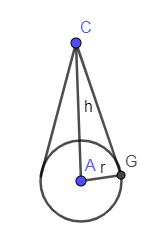Courses
Courses for Kids
Free study material
Offline Centres
MoreLast updated date: 30th Nov 2023
Total views: 279.9k
Views today: 6.79k

# The CSA of a cone is $4070c{{m}^{2}}$ and its diameter is 70cm. What is the slant height?Verified
279.9k+ views
Hint: We have given CSA (Curved Surface Area) of the cone which is equal to $4070c{{m}^{2}}$. Also, we have given the diameter of the cone. We know the relation between diameter and radius which is equal to $d=2r$ where “d” is the diameter and “r” is the radius of the cone. We know the formula for CSA of the cone which is equal to $\pi rl$ where “r” is the radius of the cone and “l” is the slant height of the cone. Equating this formula to $4070c{{m}^{2}}$ and then from this equation we will get the slant height of the cone.

In the above problem, the CSA of the cone is given as:
$4070c{{m}^{2}}$
Also, the diameter of the cone is given as:
70cm
We know the relation between the diameter and the radius of the cone as:
$d=2r$
Where “d” is the diameter and “r” is the radius of the cone.
Substituting the value of “d” as 70cm in the above equation we get,
\begin{align} & 70cm=2r \\ & \Rightarrow r=\dfrac{70}{2}cm \\ \end{align}
From this equation, we can find the value of radius using the diameter.
In the below figure, we have drawn the diagram of the cone.In the above figure, we have shown slant height by CG (let’s call it “l”).
We know the formula for the CSA of the cone which is given as follows:
$\pi rl$
In the above formula, “r” is the radius of the cone, and “l” is the slant height of the cone. Now, equating the above surface area to $4070c{{m}^{2}}$ we get,
$\pi rl=4070c{{m}^{2}}$
Substituting the value of “r” and $\pi =\dfrac{22}{7}$ in the above equation we get,
$\dfrac{22}{7}\left( \dfrac{70cm}{2} \right)l=4070c{{m}^{2}}$
In the above equation, 7 and 2 will get canceled out from the numerator and the denominator on the R.H.S of the above equation we get,
$\dfrac{11}{1}\left( 10cm \right)\left( l \right)=4070c{{m}^{2}}$
One zero and one “cm” will get canceled out from both the sides of the above equation we get,
$11\left( l \right)=407cm$
Now, dividing 11 on both the sides of the equation we get,
$l=\dfrac{407}{11}cm=37cm$
From the above, we got the value of “l” as 37 cm. Hence, we have calculated the slant height of the cone as 37 cm.

Note: To solve the above problem, you need to know the relation between diameter and radius. Also, you must know the formula for the CSA of the cone. The mistake which often occurs in this problem is that you might forget to convert the diameter into radius so make sure you won’t make this mistake.